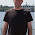## Monday, February 6, 2012

### Using apply() to create a unique id

Suppose you have a data set with two identifiers. For example, maybe you're studying the relationships among firms in an industry and you have a way to link the firms to one another. Each firm has an id, but the unique unit in your data set is a pairing of ids. Here's a stylized example of one such data set:In the example that motivated this post, I only cared that A was linked with B in my data, and if B is linked with A, that's great, but it does not make A and B any more related. In other words, the order of the link didn't matter.

In this case, you'll see that our stylized example has duplicates -- id1 = "A" and id2 = "B" is the same as id1="B" and id2 = "A" for this purpose. What's a simple way to get a unique identifier? There's an apply command for that!

Thinking of each row of the identifier data as a vector, we could alphabetize (using sort(), so c("B", "A") becomes c("A", "B")), and then paste the the resulting vector together into one identifier (paste, using collapse). I call our worker function idmaker():
idmaker = function(vec){
return(paste(sort(vec), collapse=""))
}
Then, all we need to do is use the apply command to apply this function to the rows of the data, returning a vector of results. Here's how my output looks.To get a data frame of unique links, all we need to do is cbind() the resulting vector of indices to the original data frame (and strip the duplicates). Here's some code:

co_id = apply(as.matrix(df[, c("id1", "id2")]), 1, idmaker)
df = cbind(df, co_id)
df = df[!duplicated(df[,"co_id"]),]

Here is the resulting data frame with only unique pairs.1.paste(id1, id2, sep = "") #?

2.I thought about that after posting, but it wouldn't quite do what I wanted. In particular, it wouldn't sort within each row (i.e., AB would be distinct from BA... my method removes the duplicates if you do not care about order).

3.Thanks for this apply-tutorial. I come from the sql world and each time I want to use apply I end with sql since I still have difficulties to understand apply. In sql, this problem would be solved in this way (which can also be incorporated in a function):

sqldf(paste("SELECT DISTINCT(" # elim. dupl
,"CASE WHEN id1 < id2" # sorting
," THEN id1||id2" # concatenate
," ELSE id2||id1" # concatenate
," END) AS co_id"
," FROM df"
)
)
I find it quite straightforward.

4.sort(unique(paste(df\$id1, df\$id2, sep = "")))

Greets,
Andrej

5.Andrej,

That isn't going to solve the problem my code addresses. To see why, go back to the example where id1 = "A" and id2 = "B" versus id1 = "B" and id2 = "A"

My application wants to treat these as "duplicated" in the sense that both rows in the data set indicate that "A" and "B" are linked.

If you paste it, the result for the first will be "AB" and for the second, it will be "BA" Using unique on this vector won't make the connection that "AB" and "BA" are the same for my purposes. The apply() method I put forth gives a handy way to establish the equivalence between AB and BA while also creating an index.

6.Typo:
- "... then paste the the resulting ..." -> "... then paste the resulting ..."